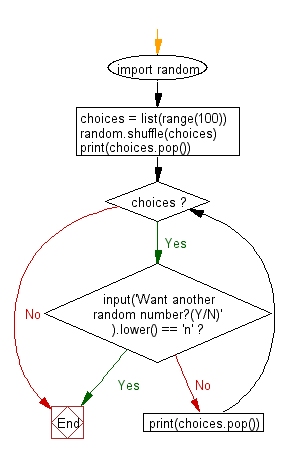﻿ Python Math: Generate a series of unique random numbers - w3resource# Python Math: Generate a series of unique random numbers

## Python Math: Exercise-81 with Solution

Write a Python program to generate a series of unique random numbers.

Sample Solution:

Python Code:

``````import random
choices = list(range(100))
random.shuffle(choices)
print(choices.pop())
while choices:
if input('Want another random number?(Y/N)' ).lower() == 'n':
break
print(choices.pop())
```
```

Sample Output:

```58
Want another random number?(Y/N)n
```

Pictorial Presentation:Flowchart:## Visualize Python code execution:

The following tool visualize what the computer is doing step-by-step as it executes the said program:

Python Code Editor:

Have another way to solve this solution? Contribute your code (and comments) through Disqus.

What is the difficulty level of this exercise?

Test your Programming skills with w3resource's quiz.

﻿

```>>> students = [{'name': 'John', 'score': 98}, {'name': 'Mike', 'score': 94}, {'name': 'Jennifer', 'score': 99}]